# Intermediate Algebra Tutorial 14

s

Intermediate Algebra
Tutorial 14: Graphing Linear Equations

WTAMU > Virtual Math Lab > Intermediate AlgebraLearning Objectives

After completing this tutorial, you should be able to:
1. Find the x- and y-intercepts of a linear function.
2. Graph a linear function using the x- and y-intercepts.
3. Graph vertical and horizontal lines.Introduction

In Tutorial 12: Graphing Equations, we went over graphing in general, learning the basics of how to graph ANY equation by plotting points.  In this tutorial we will be looking specifically at graphing lines.  The concept of using intercepts to help graph will be introduced on this page, as well as vertical and horizontal lines.  Actually, the process of graphing by plotting points and  graphing by using intercepts are essentially the same.  Intercepts are just special types of solutions, but solutions none the less.  So once we find them, we plot them just the same as any other ordered pair that is a solution.  Once we plot them, we draw our graph in the same fashion as when we had non-intercept points.  So, basically, when you graph, you plot solutions (whether they are intercept points or not) and connect the dots to get your graph.Tutorial

x-intercept

The x-intercept is where the graph crosses the x axis.

The word 'intercept' looks like the word 'intersect'.   Think of it as where the graph intersects the x-axis

With that in mind,  what value is y always going to be on the x-intercept?  No matter where you are on the x-axis, y’s value is 0,  that is a constant.  We will use that bit of information to help us find the x-intercept when given an equation.

y-intercept

If the x-intercept is where the graph crosses the x-axis where do you think the graph crosses for the y-intercept?  If you said the y-axis, you are absolutely right.

This time it is x’s value that is 0.  Any where you would cross the y-axis, x’s value is always 0.  We will use this tidbit to help us find the y-intercept when given an equation.

Below is an illustration of a graph of a linear function which highlights the x and y intercepts: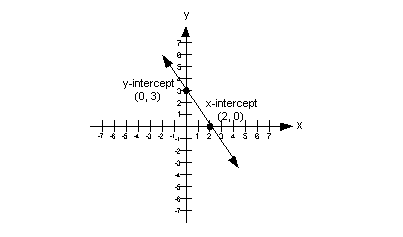In the above illustration, the x-intercept is the point (2, 0) and the y-intercept is the point (0, 3).

Keep in mind that the x- and y- intercepts are two separate points. There is only one point that can be both an x- and y- intercept at the same time, do you know what point that is?

If you said the origin (0, 0), give yourself a pat on the back.

Sketching a Graph
Using Intercepts

Step 1:  Find the x- and y- intercepts.

You find the x-intercept by plugging in 0 for y and solving for x.

You find the y-intercept by plugging in 0 for x and solving for y.

Step 2:  Find at least one more point.

You do this by plugging in ANY value(s) for x and finding it's corresponding y value.

This is just like we showed you in Tutorial 12: Graphing Equations.

Step 3:  Plot the intercepts and point(s) found in steps 1 and 2.

Remember that intercepts are points on the graph, too.  They are plotted just like any other point.

Step 4:  Draw the graph.

The graph of a linear function is a straight line.Example 1:  Graph each linear function by finding the x- and y- intercepts.   y= 5 - 3x

Step 1:  Find the x- and y- intercepts.

Let’s first find the x-intercept
What value are we going to use for y
You are correct if you said y = 0.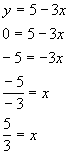*Find x-int. by replacing y with 0
*Inverse of add 5 is sub. 5

*Inverse of mult. by -3 is div. by -3

The x-intercept is (5/3, 0).

Next, we will find the y- intercept.
What value are we going to plug in for x
If you said x = 0 you are right.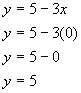*Find y-int. by replacing x with 0

The y-intercept is (0, 5)

Step 2:  Find at least one more point.

We can plug in any x value we want as long as we get the right corresponding y value and the function exists there.

Let’s put in an easy number  x = 1: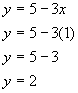*Replace x with 1

So the ordered pair (1, 2) is another solution to our function.

Note that we could have plugged in any value for x: 5, 10, -25, ...,  but it is best to keep it as simple as possible.

The solutions that we found are:

x y (x, y) 5/3 0 (5/3, 0) 0 5 (0, 5) 1 2 (1, 2)

Step 3: Plot the intercepts and point(s) found in steps 1 and 2.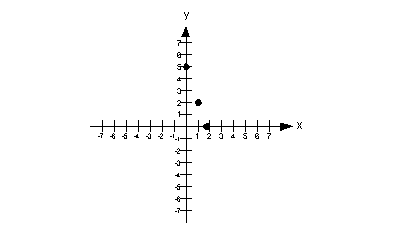Step 4:  Draw the graph.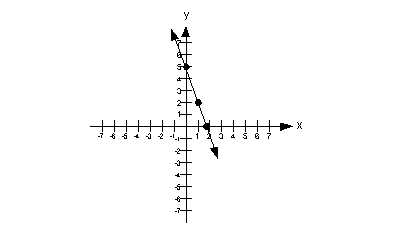Example 2:  Graph each linear function by finding the x- and y- intercepts.   -3x = y

Step 1:  Find the x- and y- intercepts.

Let’s first find the x-intercept
What value are we going to use for y
You are correct if you said y = 0.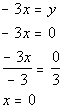*Find x-int. by replacing y with 0
*Inverse of mult. by -3 is div. by -3

The x-intercept is (0, 0).

Next, we will find the y- intercept.
What value are we going to plug in for x
If you said x = 0, you are right.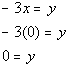*Find y-int. by replacing x with 0

The y-intercept is (0, 0)

Hey, look at that, we ended up with the exact same point for both our x- and y-intercepts.  As mentioned above, there is only one point that can be both an x- and y- intercept at the same time, the origin (0, 0).

Step 2:  Find at least one more point.

Since we really have found only one point this time, we better find two additional solutions so we have a total of three points.

We can plug in any x value we want as long as we get the right corresponding y value and the function exists there.

Let’s put in an easy number x = 1: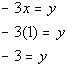*Replace x with 1

So the ordered pair (1, -3) is another solution to our function.

Let’s put in another easy number x = -1: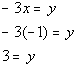*Replace x with -1

So the ordered pair (-1, 3) is another solution to our function.

Note that we could have plugged in any value for x: 5, 10, -25, ...,  but it is best to keep it as simple as possible.

The solutions that we found are:

x y (x, y) 0 0 (0, 0) 1 -3 (1, -3) -1 3 (-1, 3)

Step 3: Plot the intercepts and point(s) found in steps 1 and 2.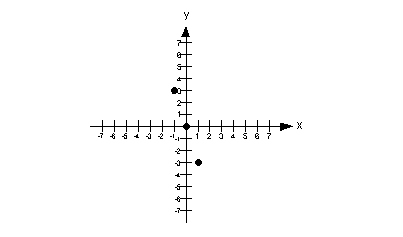Step 4:  Draw the graph.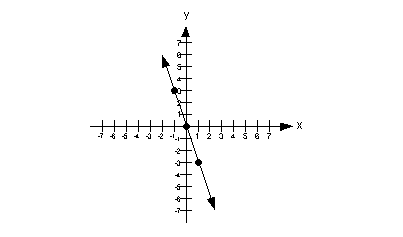Vertical Lines

x = c

If you have an equation x = c, where c is a constant, and you are wanting to graph it on a two dimensional graph, this would be a vertical line with x-intercept of (c, 0).

Even though you do not see a y in the equation, you can still graph it on a two dimensional graph.  Remember that the graph is the set of all solutions for a given equation.  If all the points are solutions then any ordered pair that has an x value of c would be a solution.  As long as  x never changes value,  it is always c, then you have a solution.  In that case, you will end up with a vertical line.

Below is an illustration of a vertical line x = c: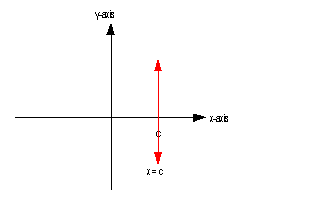Horizontal Lines

y = c

If you have an equation y = c, where c is a constant, and you are wanting to graph it on a two dimensional graph, this would be a horizontal line with y- intercept of (0, c).

Even though you do not see an x in the equation, you can still graph it on a two dimensional graph.  Remember that the graph is the set of all solutions for a given equation.  If all the points are solutions then any ordered pair that has an y value of c would be a solution.  As long as y never changes value,  it is always c, then you have a solution.  In that case, you will end up with a horizontal line.

Below is an illustration of a horizontal line y = c: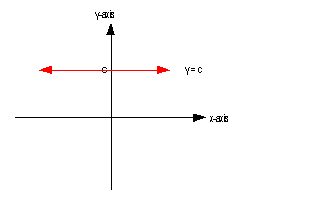Example 3:  Graph the linear equation y = 4.

It looks like it fits the form y = c.
With that in mind, what kind of line are we going to end up with?
Horizontal.

Note how the directions did not specify that we had to use intercepts to do our graph.  Any time you take a math test or do homework, make sure that you follow directions carefully.  If it specifies a certain way to do a problem, then you need to follow that plan (like in the above examples 1 and 2).  If it does not specify, like in this example, then you can use what ever “legitimate” way works to get the job done.

Since this is a special type of line, I thought I would talk about steps 1 and 2 together.

It doesn’t matter what x is, y is always 4.  So for our solutions we just need three ordered pairs such that y = 4.

Note that the y-intercept (where x = 0) is at (0, 4).

Do we have a x-intercept? The answer is no.  Since y has to be 4, then it can never equal 0, which is the criteria of an x-intercept.  Also, think about it, if we have a horizontal line that crosses the y-axis at 4, it will never ever cross the x-axis.

So, some points that we can use are (0, 4), (1, 4) and (2, 4).  These are all ordered pairs that fit the criteria of y having to be 4.

Of course, we could have used other solutions, there are an infinite number of them.

The solutions that we found are:

x y (x, y) 0 4 (0, 4) 1 4 (1, 4) 2
4 (2, 4)

Step 3:  Plot the intercepts and point(s) found in steps 1 and 2.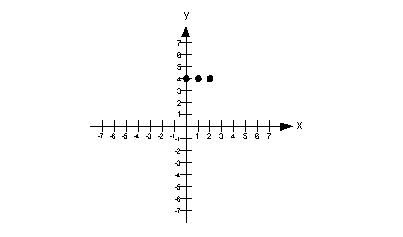Step 4:  Draw the graph.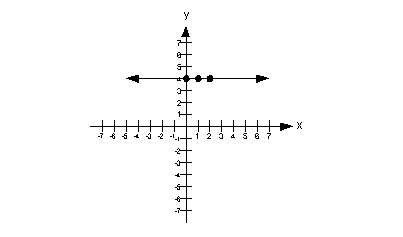Example 4:  Graph the linear equation x + 3 = 0.

Note how if we subtract 3 from both sides, we can write this as x = -3, which means it can be written in the form x = c
So, what type of line are we going to end up with?
Vertical.

Since this is a special type of line, I thought I would talk about steps 1 and 2 together.

It does not matter what y is, as long as x is -3.

Note that the x-intercept is at (-3, 0).

Do we have a y-intercept?  The answer is no.  Since x can never equal 0, then there will be no y-intercept for this equation.

Some points that would be solutions are (-3, 0), (-3, 1), and (-3, 2).

Again, I could have picked an infinite number of solutions.

The solutions that we found are:

x y (x, y) -3 0 (-3, 0) -3 1 (-3, 1) -3 2 (-3, 2 )

Step 3:  Plot the intercepts and point(s) found in steps 1 and 2.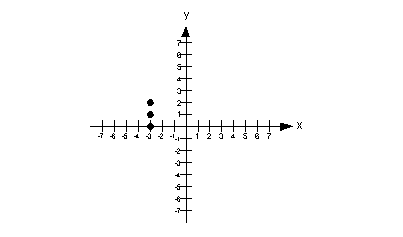Step 4:  Draw the graph.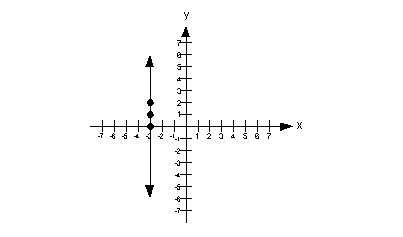Practice Problems

These are practice problems to help bring you to the next level.  It will allow you to check and see if you have an understanding of these types of problems. Math works just like anything else, if you want to get good at it, then you need to practice it.  Even the best athletes and musicians had help along the way and lots of practice, practice, practice, to get good at their sport or instrument.  In fact there is no such thing as too much practice.

To get the most out of these, you should work the problem out on your own and then check your answer by clicking on the link for the answer/discussion for that  problem.  At the link you will find the answer as well as any steps that went into finding that answer.Practice Problems 1a - 1b: Graph each linear function by finding x- and y-intercepts.

1a.  2x - 3y = -6
1b.  x = 3yPractice Problems 2a - 2b: Graph each linear equation.

2a.  x = 4
2b.  y + 5 = 0Need Extra Help on these Topics?

The following is a webpage that can assist you in the topics that were covered on this page:

http://www.purplemath.com/modules/intrcept.htm
This website goes over x- and y-intercepts.

Go to Get Help Outside the Classroom found in Tutorial 1: How to Succeed in a Math Class for some more suggestions.Last revised on July 3, 2011 by Kim Seward.